• 2018-10-25 12:46:56

拉格朗日乘数法：

构造拉格朗日方程：设给定二元函数z=ƒ(x,y)和附加条件φ(x,y)=0，为寻找z=ƒ(x,y)在附加条件下的极值点，先做拉格朗日函数，其中λ为参数。

求分别对应的偏导，=0，求出极值点，运算比较

更多相关内容
• 约束条件多元函数极值的充分条件问题通常是采用二阶微分法来判断,该方法原理虽然简单,但计算量大,尤其是随着变量和约束条件个数的增加,要计算出d2 L并判断出其符号就显得更加困难而不可行。文章用Lagrange乘数法...
• 约束条件多元函数条件极值的充分条件，杨斌，沙春宏，在等约束条件下用 乘数法、多元隐函数求导法以及有条件极值化无条件极值的方法推导证明了多元函数极值的充分条件，并给出易于计�
• 请教，约束条件多元函数最小值的求解 请假各位高手，我的代码的第二个函数，就是那个二元函数g，目前的求解是没有任何约束的，我要在进行求解时，加入约束t1和t2都大于零，并且t1小于t2来进行求解，之前，我参照了...请教，约束条件下多元函数最小值的求解

请假各位高手，我的代码的第二个函数，就是那个二元函数g，目前的求解是没有任何约束的，我要在进行求解时，加入约束t1和t2都大于零，并且t1小于t2来进行求解，之前，我参照了一些教材，但是结果错的很离谱，希望各位高手指点，该如何做。

另外还有一个问题。t0设置成其他参数，就报错了，请问它的设置有什么讲究。

a=3000;

A=50;

c=40;

p=50;

h=3;

b=20;

ie=0.02;

ip=0.04;

B=10;

vb=0:0.05:0.7;

i=1;

diff = 0;

result = zeros(1,15);

ans = zeros(2,15);

%f=@(x)m*(x^2)+n*x+a*c+A/x;

%g=@(t)m1*(t(1)^2)+n1*t(1)+B*b*t(2)+a*B+c*a+c*b*t(2)+m2*(t(1)^2)/t(2)+A/t(2)-a*B*t(1)/t(2)+n2*(t(1)^3)/t(2);

for vb = 0:0.05:0.7;

m = 2/3*b*h+c*b*(1.-vb)*ip-1/3*p*b*ie;

n = b*c+1/2*a*h-1/2*p*a*ie+c*a*(1.-vb)*ip;

m1 = (p*b*ie.*((vb.^2)-1)+p*b*ie*(c/p-(vb.^2)));

n1 = (p*a*ie.*(-1.+vb)+p*a*ie.*(c/p-vb));

m2 = (a*ie.*vb.*(p.*vb-c)-(a*p*ie.*((vb.^2)-(0.5)))+((0.5*a*h)-(b*B)));

n2 = (a*ie.*vb.*(p.*(vb.^2)-c)-p*b*ie.*((vb.^3)-(2/3))+2/3*b*h);

f=@(x)m*(x^2)+n*x+a*c+A/x;

[x(i),y(i)]=fminbnd(f,0,5);

g=@(t)m1*(t(1)^2)+n1*t(1)+B*b*t(2)+a*B+c*a+c*b*t(2)+m2*(t(1)^2)/t(2)+A/t(2)-a*B*t(1)/t(2)+n2*(t(1)^3)/t(2);

t0 = [-1,1];

[t,fval]=fminunc(g,t0);

result(i)=fval;

ans(1,i)=t(1);

ans(2,i)=t(2);

i=i+1;

end

ans,x,y,fval

vb = 0:0.05:0.7;

plot(vb,y)

hold on

vb=0:0.05:0.7;

result;

plot(vb,result)

展开全文• 这里是比较奇怪的，因为这道题目的约束条件是x，但是求的最值是(a+1)b, 这道题目的正常做法就是由不等式关系恒成立，推出a和b必须满足的条件，然后再来求(a+1)b的最值，这样有点模糊。但幸好f(x)的形式比较简单。 ...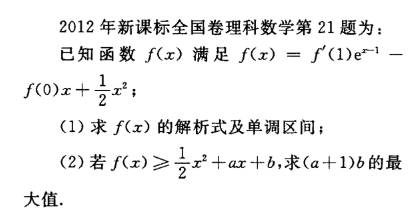这道题目编的比较好，第一问就构造的特别巧妙

（1） f(x)' = f‘(1)e^(x-1) - f(0) + x                                          f'(1) = f'(1) - f(0) + 1,        f(0)=1

f(x) = f'(1)e^(x-1) - f(0)x + 1/2x^2                                 f(0) = f'(1) e^(-1)               f'(1) = e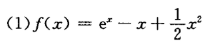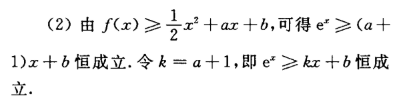这里是比较奇怪的，因为这道题目的约束条件是x，但是求的最值是(a+1)b, 这道题目的正常做法就是由不等式关系恒成立，推出a和b必须满足的条件，然后再来求(a+1)b的最值，这样有点模糊。但幸好f(x)的形式比较简单。

f(x)= e^x - (a+1)x - b >=0  求(a+1)b的最大值。 这里想到规范化的技巧能够减小计算量令k=a+1

f(x)= e^x - kx-b >=0, 求kb的最大值。

f‘(x) = e^x -k ,    当k<0 时，f'(x) > 0 f(x)>=f(0)>=0     =>b>=0

当k>0时，f(x)>=f(ln(k)) = k-klnk-b>=0

上面的题目等价于k-klnk>=b时，求bk的最大值

bk<=k^2-k^2ln(k)

令f(k)=k^2-k^2ln(k)

这道题目其实是用极值点防缩，然后巧妙的实现了消元，其中第二步依赖于b刚好可以消元。

已知b-k+klnk<=0, 求-bk的最小值，可以尝试用拉格朗日数乘法+KKT条件做

构造L(b，k） = -(bk) + λ(b-k+klnk)

L对b求导  -k + λ = 0,  λ=k

L对k求导  -b + λ(-1+1+lnk)=0        -b + λln(k)=0

两个等式解得  kln(k) = b           2klnk - k =0， 解得k=0，或者k=exp(1/2)   b=exp(1/2)*1/2,

bk的最大值时exp(1)/2， 容易严重满足KKT条件

实际上，这道题目一上来直接套用凸优化的拉格朗日乘数法+KKT条件也是可以做出来的，其中关于x的偏导数为0的方程就是第一个部消去的步骤。

这道题目还可以用几何意义做出来，这也是拉格朗日乘数法和KKT条件的几何意义。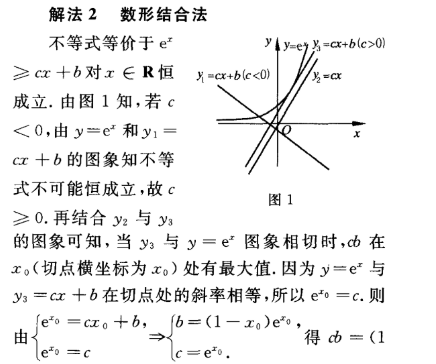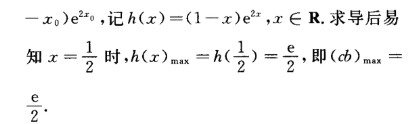附录KKT条件的结论。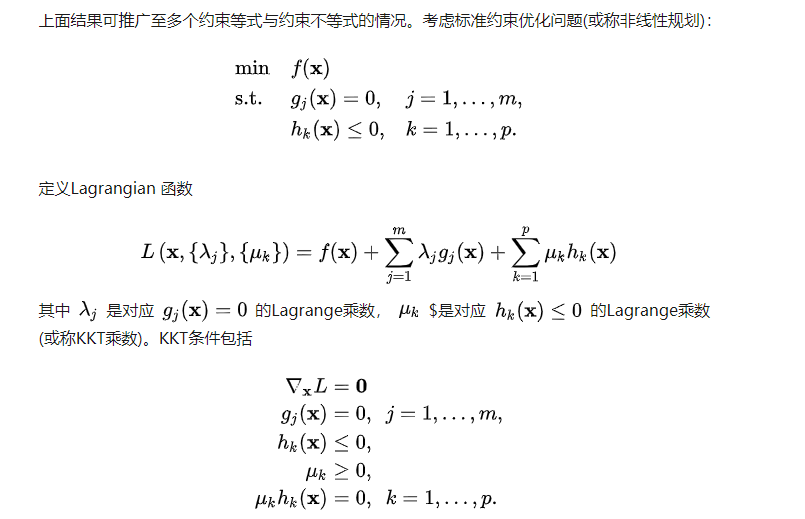展开全文•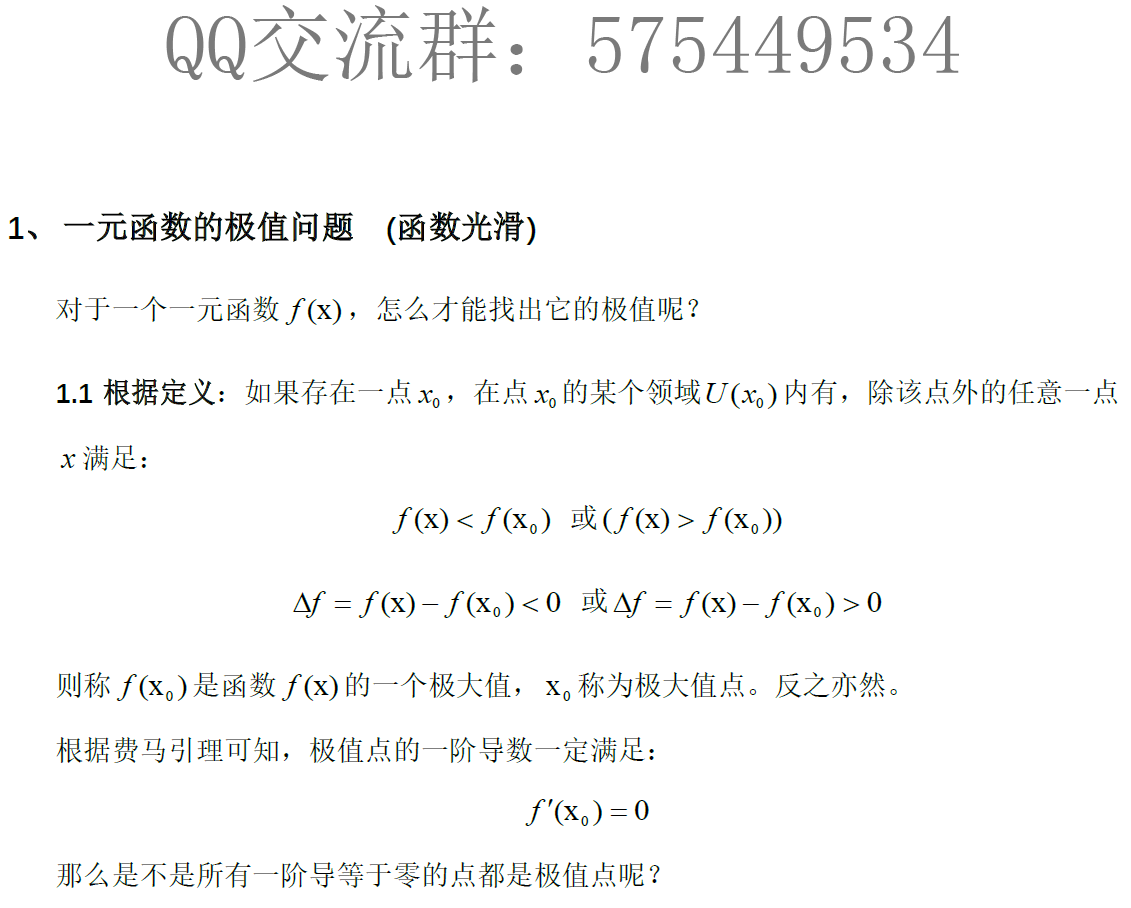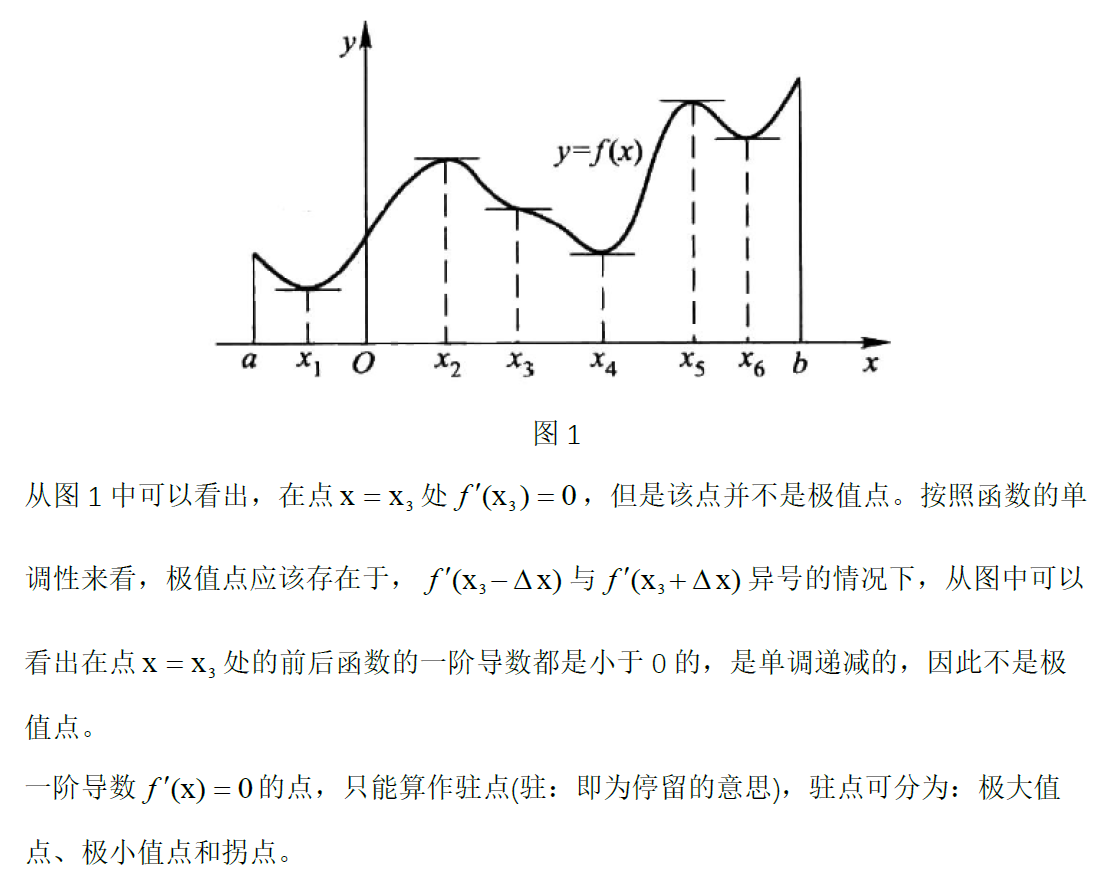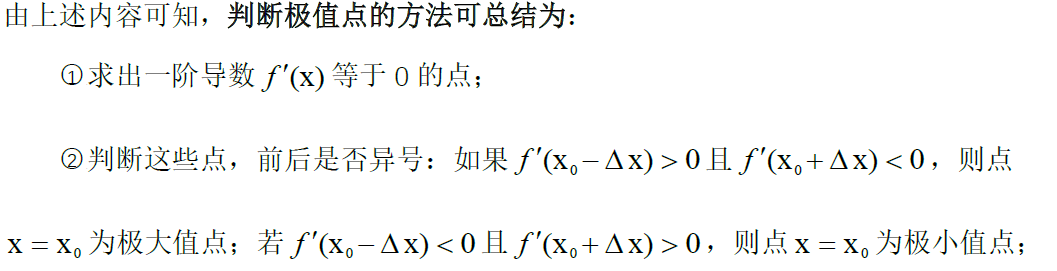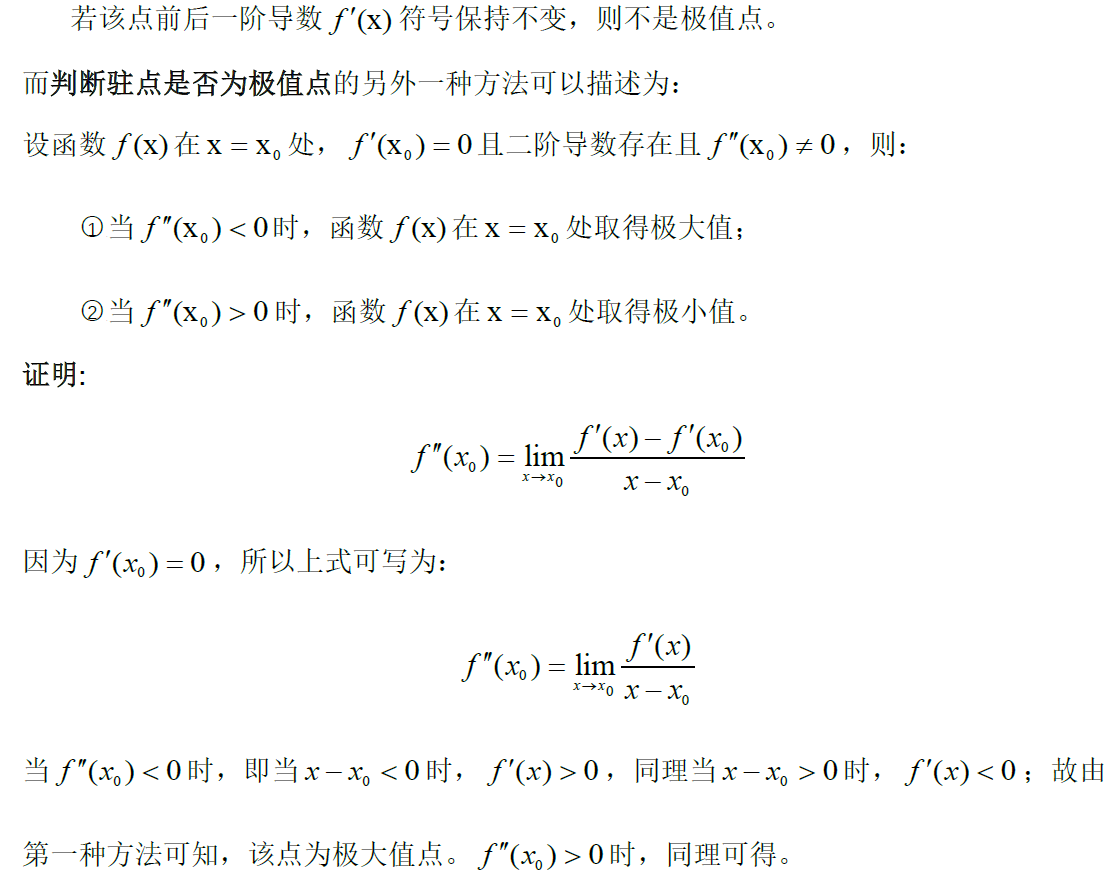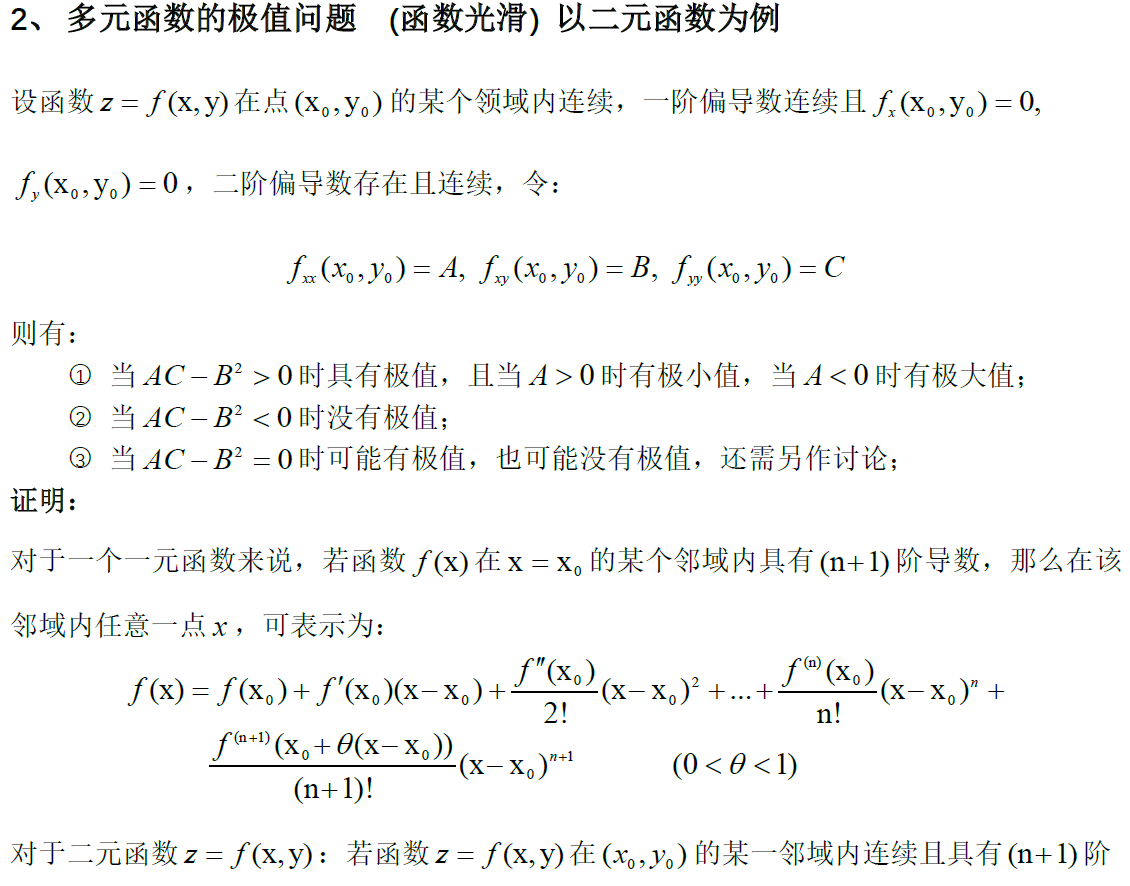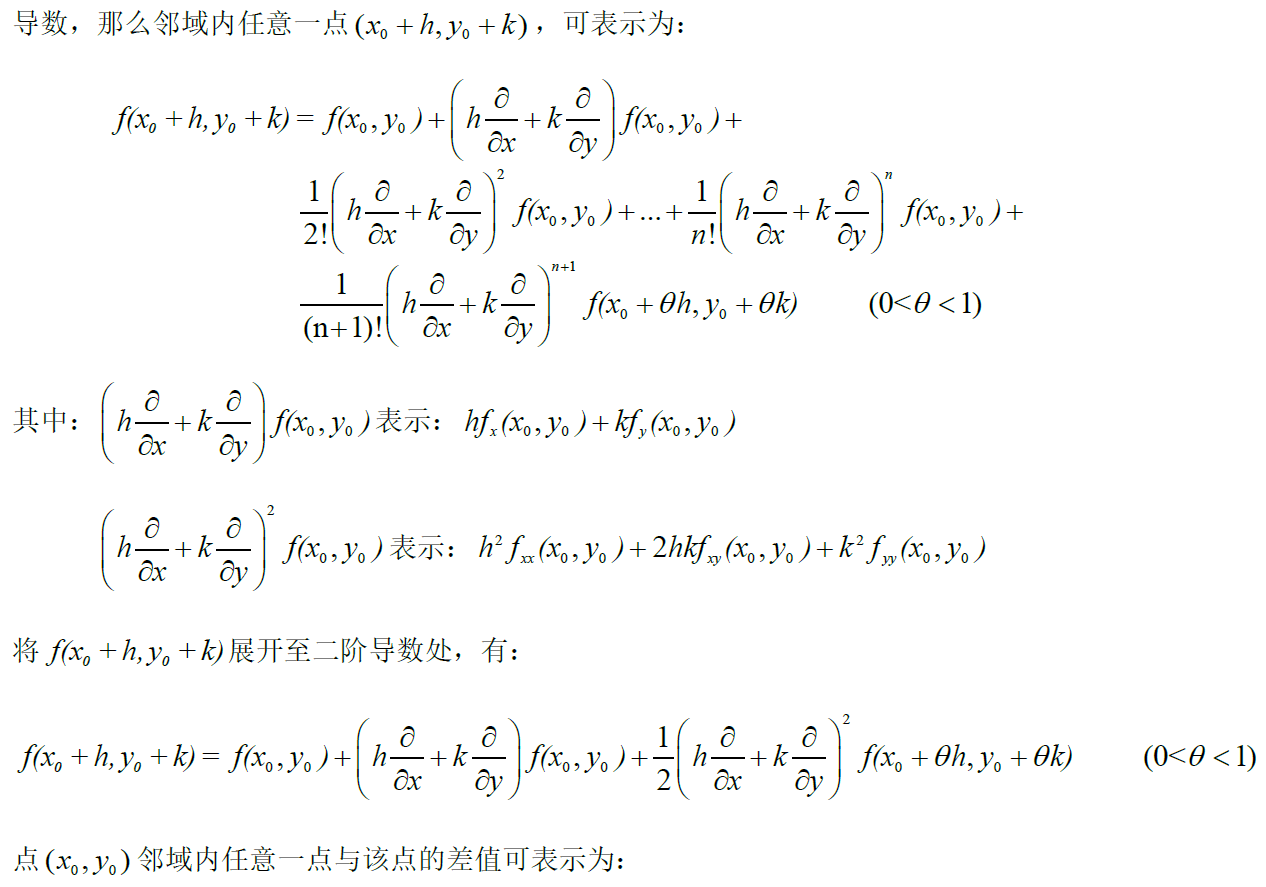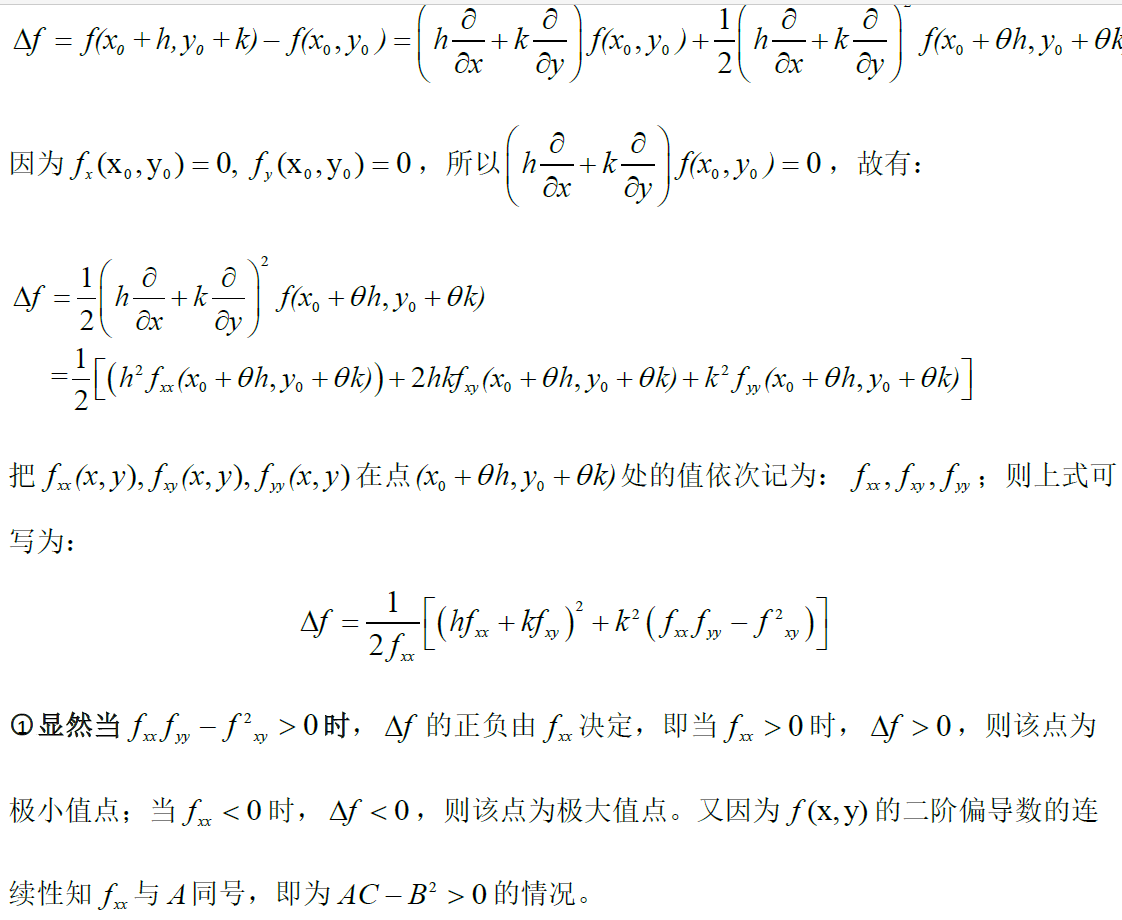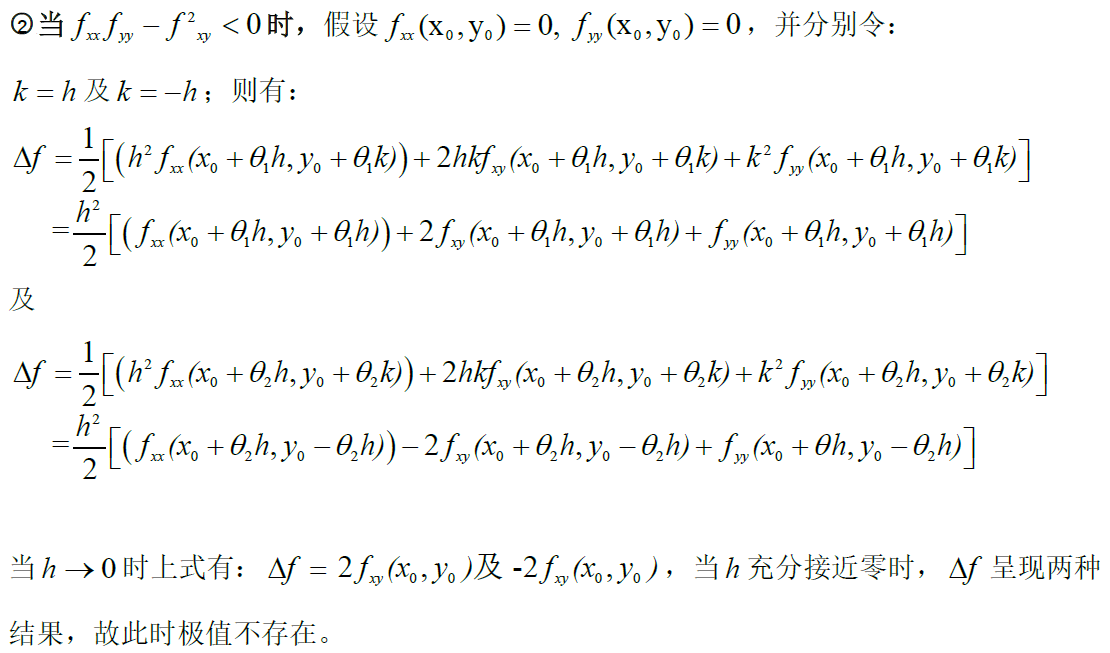展开全文一元函数
• 20.2.2 多元函数约束优化在MATLAB中，对形如 的多元函数约束优化问题提供了下列求解函数。(1)x= fminunc(fun, X0)，或x=fminsearch(fun, X0)。(2)x= fminunc(fun, X0, options)，或x=fminsearch(fun,X0, options...
• 拉格朗日乘数法（Lagrange multiplier，以数学家约瑟夫·拉格朗日命名），在数学中的最优化问题中，是一种寻找多元函数在其变量受到一个或多个条件约束时的极值的方法。这种方法可以将一个有 nnn 个变量与 kkk 个...拉格朗日乘子法 求极值 拉格朗日
• 多元函数f(x,y)=x3-y3+3x2+3y2-9x的极值 %% clear f=@(x)x(1)^3-x(2)^3+3*x(1)^2+3*x(2)^2-9*x(1); g=@(x)-f(x); [xmin,ymin]=fminunc(f,rand(2,1)) %求极小值点 [xmax,ymax]=fminsearch(g,rand(2,1)) %求极大值...matlab
• 转化为无条件极值在讨论多元函数极值问题时，如果遇到除了在定义域中寻求驻点(可能的极值点)外，对自变量再无别的限制条件，我们称这类问题为函数的无条件极值。如求的极值，就是无条件极值问题。然而在实际中，...
• 采用Armijo线搜索的牛顿法求解多元非线性函数结果matlab代码
• 看到多元函数条件极值的题目，常用拉格朗日乘数法对号入座。但有时候如坐针毡，因为这种看似万能的方法计算量太大了。解方程解的生无可恋是常态。所以我总结了一些解条件极值的小技巧，希望对大家有所帮助。 总的来...算法
• 用遗传算法求解带约束多元函数极值问题（Java实现） 1.问题描述 针对如下问题，设计遗传算法进行求解： 2.Java源代码 GA.java package GA; import java.util.Random; class GA { public static final int var...java 算法
• ## 多元函数极值问题

千次阅读 2020-05-17 22:11:45
多元函数极值问题 可以分为以下三个方面 无约束极值问题 等式约束条件极值问题 不等式约束条件极值问题 无约束条件多元函数极值算法
• 利用MATLAB求多元函数的极值分两种情况，(1)无约束条件；(2)有约束条件。(2)有约束条件下求极小值的方法:假设多变量非线性函数的数学模型为min f(x)c(x)<=0ceq(x)=0A·x<=bAeq·x<=x<=beqlb<=x<=...
• 模拟退火算法解多元函数 题目： F(x)=11.16386−0.0903x1−0.1487x2−0.0664x3+0.09074x4−2.452∗10−4x1x2+6.228∗10−5x1x3+2.457∗10−3x1x4+3.8688∗10−3x2x3−6.471∗10−3x2x4−1.451∗10−3x3x4F(x)=11....模拟退火算法
• 数学竞赛辅导函数恒成立问题专题训练1. 已知不等式 x + m ≤ 0 在区间［2,3］上恒成立，求实数m 的取值范围。 2 x 2 -92．设函数f (x ) =2x 3+3ax 2+3bx +8c 在x =1及x =2时取得极值． (Ⅰ)求a 、b 的值；(Ⅱ)若对于...
• 1. 引言我们在中学的时候学过一元二次函数，求解时引入一个求根公式，代入公式就可以得到不同的根，假如想计算一个高次方程的解，我们还能推导出求根公式吗？伽罗瓦在群论中证实，五次及以上多项式方程没有显示表达...
• 多元函数的极限与连续 1，对于f()函数内部的变量数目>1时的处理方法： 一般情况是 可以运用多次变量代还来转换原函数来使得原f 函数内部变量尽量简洁或具有特性，同时也可以将新的函数f与和该f函数内变量相关的项...
• 多元非线性目标函数求解之目标函数约束条件如何写
• 函数分段点极限。 3. 偏导数 利用定义法判定存在。 4. 可微 写出全增量Δz=f(x0+Δx,y0+Δy)−f(x0,y0)\Delta z=f(x_0+ \Delta x,y_0+\Delta y)-f(x_0,y_0)Δz=f(x0​+Δx,y0​+Δy)−f(x0​,y0​) 写出线性增量A...高等数学 思维导图 数学
• ## 多元函数的极值

万次阅读 2018-08-18 09:59:26
多元函数的极值 定义 z=f(x,y) (x,y)∈∈\inD,M0(x0,y0)∈D(M0是D的内点),U(M0,δ(域))⊂DM0(x0,y0)∈D(M0是D的内点),U(M0,δ(域))⊂DM_0(x_0,y_0)\in D(M_0是D的内点) ,U(M_0,\delta(域))\subset D 若f(x0,y0x...拉格朗日乘子法
• 本篇中我们主要总结一下多元函数的代数应用——多元函数找寻极值点。 在开始之前，先做一个回顾，还是老规矩，我们以一元函数的极值比对多元函数的这一部分内容。 回顾 一元函数极值的定义 在y=f(x)上一点x0的去心...
• 利用MATLAB求多元函数的极值分两种情况，(1)无约束条件；(2)有约束条件。(2)有约束条件下求极小值的方法:假设多变量非线性函数的数学模型为min f(x)c(x)<=0ceq(x)=0A·x<=bAeq·x<=x<=beqlb<=x<=...
• 如何用matlab或lingo求解多元函数的最小值?求最优成本 minC，minC=X[ ]*P[ ]，价格P固定，重量X变动为整数，如无法得出整数解，有小数解也可。X[]=[x1,x2,x3,x4,x5,x6,x7,x8,x9,x10,x11,x12]P[]=[6900,5330,4800,...
• 在约束下，求二元方程组X,Y极值问题 采用Matlab中的fmincon函数求多元函数约束极值问题 当问题变为多目标极值问题时可以采用gamultiobj和fminimax函数matlab
• §8.8 多元函数极值及其求法 一、多元函数的极值 1、多元函数极值定义 设函数在点的某个邻域内有定义,对该邻域内异于的点,如果都适合不等式 则称函数在点取极大值； 如果都适合不等式 则称函数在点取极小...
• 一、多元函数的极限与连续 （1）多元函数的二重极限 1、证明函数的极限是否存在： (1) 定义证明：与一重极限相似，x之间的距离无限逼近变为点（x，y）与聚点的距离无限逼近， 即当满足式子 0<<任意正数的...
• 利用MATLAB求多元函数的极值分两种情况，(1)无约束条件；(2)有约束条件。(2)有约束条件下求极小值的方法:假设多变量非线性函数的数学模型为min f(x)c(x)<=0ceq(x)=0A·x<=bAeq·x<=x<=beqlb<=x<=...
• 一、多元函数的复合函数求导法则 1、链式求导法 2、全微分形式不变性 全微分运算的四则运算性质 3、隐函数微分法 3.1 一个方程的情形 经典例题...学习...